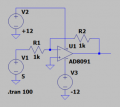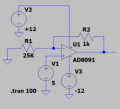# calculate resistor values

#### pinkyponky

Joined Nov 28, 2019
152
Hi all,

In my circuit, I need to use the unity gain amplifier by using the inverting amplifier as a voltage inverter. But, I don't know how to choose/calculate the Rf and Rin resistor values for the inverting amplifier. In my case, The output current of the inverting amplifier maximum 20mA. So, Please, could you help me what range of resistor values need to be chosen?.

#### ericgibbs

Joined Jan 29, 2010
13,825
hi pp,
Inverting OPA Gain = -Rfb/Rin,
so if Rin = 4k7 and Rfb =4k7 Gain = -1

E

#### Ian0

Joined Aug 7, 2020
3,463
Rin will be the input impedance of the circuit. Choose a value that the source can easily drive. For unity gain the feedback resistor is the same as the input resistor as @ericgibbs said.
The op amp has to drive the feedback resistor, so choose a value so that Vout/Rfb << 20mA.

#### pinkyponky

Joined Nov 28, 2019
152
hi pp,
Inverting OPA Gain = -Rfb/Rin,
so if Rin = 4k7 and Rfb =4k7 Gain = -1

E
Thank you Eric.

Is there any description or calculations?, why you chosen Rin = Rfb = 4k7?. And also I have seen that the people use to use the 1k/4k7/10k resistors. Why these resistors are most popular?.

#### Ian0

Joined Aug 7, 2020
3,463
If you have 10V output, it will take 10mA to drive the 1k feedback resistor. Your op-amp can only drive 20mA, so there is only 10mA left to drive any external load. So, it‘s not a problem unless you have a load of <1k.

I wonder if 4.7k is so popular because it was de facto standard pull-up resistor for TTL so everyone had a drawer full of them.

•anhnha

#### ericgibbs

Joined Jan 29, 2010
13,825
hi pp,
Post a circuit diagram, then we can follow what you are trying to do, rather than guessing.E

#### pinkyponky

Joined Nov 28, 2019
152
hi pp,
Post a circuit diagram, then we can follow what you are trying to do, rather than guessing.E
Hi Eric,

The chosen Rin and Rf resistors are shown in the below circuits. And also, I would like to know how to calculate the output current of AD8091 ?.

First circuit is designed to invert the voltage.
Second circuit is designed to increase the output voltage.
But, the output current of the both the circuits shall be maximum 20mA.#### ericgibbs

Joined Jan 29, 2010
13,825
Second circuit is designed to increase the output voltage.
hi pp,
The 2nd circuit is incorrect, if I follow your thinking.? the 25k and 1k should be switched around.

BUT you cannot get a Gain of 25 with a 5Vdc input.

I will post a circuit that may suit.
E

#### ericgibbs

Joined Jan 29, 2010
13,825
hi pp,
What voltage do you want from the Vout when the Load current is 30mA.?
E

#### Ian0

Joined Aug 7, 2020
3,463
Hi Eric,

The chosen Rin and Rf resistors are shown in the below circuits. And also, I would like to know how to calculate the output current of AD8091 ?.

First circuit is designed to invert the voltage.
Second circuit is designed to increase the output voltage.
But, the output current of the both the circuits shall be maximum 20mA.

View attachment 246056

View attachment 246057
The second circuit DOES increase the output voltage, but not by much.
$$Gain=1+\frac{R2}{R1}$$
which works out to be 1.04.

The output current depends on the load resistance which you have not shown.
$$I_{out}=\frac{V_{out}}{R2}+\frac{V_{out}}{R_{load}}$$

#### pinkyponky

Joined Nov 28, 2019
152
hi pp,
The 2nd circuit is incorrect, if I follow your thinking.? the 25k and 1k should be switched around.

BUT you cannot get a Gain of 25 with a 5Vdc input.

I will post a circuit that may suit.
E
The second circuit I have increased the output voltage to 5.2V with the gain of 1.04. I think it's correct.

#### pinkyponky

Joined Nov 28, 2019
152
hi pp,
What voltage do you want from the Vout when the Load current is 30mA.?
E
I want 5.2V when the output current is 20mA.

#### pinkyponky

Joined Nov 28, 2019
152
The second circuit DOES increase the output voltage, but not by much.
$$Gain=1+\frac{R2}{R1}$$
which works out to be 1.04.

The output current depends on the load resistance which you have not shown.
$$I_{out}=\frac{V_{out}}{R2}+\frac{V_{out}}{R_{load}}$$
Yes, it will increase the voltage from 5V to 5.2V with the gain of 1.04.

#### pinkyponky

Joined Nov 28, 2019
152
hi,
Look at this option.
As set up 5.2V out for -5.2Vin, test load is 20mA.
You can get higher currents if required,

E
View attachment 246061

I have a question that, shall we not use the R1 and R2 resistors = 1kohm?.

#### Ian0

Joined Aug 7, 2020
3,463
The Ad8091 is capable of 45mA output current, so 20mA output plus 10mA in the feedback resistor (if you use 1k resistors) won’t be a problem.
However, it has an Absolute Maximum power supply voltage of 12.6V and you have 24V. That will be a problem.

#### ericgibbs

Joined Jan 29, 2010
13,825
hi pp,
Exactly how is this 20/30mA at 5.2V going to be used.??

What is the 20/30mA driving into.??

E